Ask your WordPress questions! Pay money and get answers fast!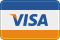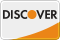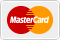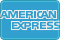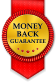Get age from birthdate function WordPress

• SOLVED

Code below generates the author birthdate.

``` if (\$term_meta['birth']) { echo '<li><span class="custom-text">Birth:</span>&nbsp;','<span class="author-text">'.\$term_meta['birth'].'</span></li>'; } ```

I need a function that will get the author age from the birthdate using the variable \$term_meta['birth'] which is where the birthdate is stored.

2013-12-12

```\$birthday = \$term_meta['birth']; \$interval = \$birthday->diff(new DateTime); echo \$interval->y;```

Full code

``` if (\$term_meta['birth']) { \$birthday = \$term_meta['birth']; \$interval = \$birthday->diff(new DateTime); echo '<li><span class="custom-text">Birth:</span>&nbsp;','<span class="author-text">'.\$interval->y.'</span></li>'; } ```

Function:

```function getAge(\$birthday) { \$birthday = new DateTime(\$birthday); \$interval = \$birthday->diff(new DateTime); return \$interval->y; }```

So the code will be

if (\$term_meta['birth']) {
echo '<li><span class="custom-text">Birth:</span>&nbsp;','<span class="author-text">'.getAge(\$term_meta['birth']).'</span></li>'; }

Hi Fahd.
What would be the best way to add the echo to an if statement?
I tried this.

Line 76`if (\$term_meta['birth']) { echo '<li><span class="custom-text">Birth:</span>&nbsp;','<span class="author-text">'\$interval->y;'</span></li>'; }`

no luck I'm getting this.
Parse error: syntax error, unexpected T_VARIABLE, expecting ',' or ';' in /home/quotetwe/public_html/wp-content/themes/Nexus/index.php on line 76

ok just seen your other posts let me try. thank you.

OK, let me know if any issues. Also whats your date format? I remember writing a detailed blog post about this but can't find the link as I killed my old blog :(

M,D,Y as far as I know. Works like a charm! Thank you my man. If you would like to check out how far this little project has come here is the url http://quotetweet.com/authors/adam-sandler/

Adam Sandler is the best, wish you success for the project.

```function get_age( \$birthdate ) { // Explode the date into useful variables... adjust as needed for the format you are storing the date in. // This assumes you are storing as YYYY-mm-dd list( \$year, \$month, \$date ) = explode( '-', \$birthdate ); // Calculate the differences \$yeardiff = date( 'Y' ) - \$year; \$monthdiff = date( 'm' ) - \$month; \$daydiff = date( 'd' ) - \$day; // Check if the birthday has occurred yet this year if( \$daydiff < 0 || \$monthdiff < 0 ) { \$yeardiff--; } return \$yeardiff; }```